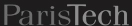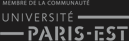Fermer X

# Dam Optimal Management under Uncertainty

P. Carpentier, J.-P. Chancelier, M. De Lara and V. Leclère
(last modiﬁcation date: April 11, 2018)
Version pdf de ce document
Version sans bandeaux

### Contents

Abstract

In this practical work, you will play the role of a dam manager. You will try to maximize the mean intertemporal payoﬀ obtained by selling hydropower produced by water releases, when the water inﬂows (rain, snowmelt, outﬂows from upper dams) are supposed to be random and the energy prices deterministic. You will propose diﬀerent water turbinated policies and you will compute their expected payoﬀ. Then, you will compute the optimal payoﬀ and display an optimal policy. At last, you will consider diﬀerent variations around this problem: highlighting the information structure inﬂuence by comparing the “decision-hazard” and the “hazard-decision” settings, evaluating a “fair ﬁnal value of the water” or testing robustness with respect to inﬂow probability distribution.

### 1 Problem statement and data

We consider a dam manager intending to maximize the intertemporal payoﬀ obtained by selling power produced by water releases, when the water inﬂows (rain, snowmelt, outﬂows from upper dams) are random and the energy prices are supposed to be deterministic.

#### 1.1 Dam dynamics

We model the dynamics of the water volume in a dam by a function f deﬁned as follows1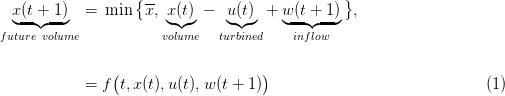where

• time t 𝕋 = {ti,ti + 1,,tf} is discrete (months in this case), and t denotes the beginning of the period [t,t + 1[; we call ti the initial time and tf the horizon;
• x(t) volume of water in the dam at the beginning of period [t,t + 1[, belonging to a ﬁnite set 𝕏 [x,x], where x (resp. x) is the minimum (resp. maximum) dam volume;
• u(t) turbinated outﬂow volume during [t,t + 1[, decided at the beginning of the time period [t,t + 1[, and belonging to a ﬁnite set 𝕌 [u,u], where u (resp. u) is the minimum (resp. maximum) volume which can be turbinated by time unit; we assume that the minimum value u is equal to zero: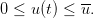(2)

• w(t) inﬂow water volume (rain, snowmelt, etc.) during the time period [t1,t[, belonging to the ﬁnite set 𝕎(t) [w(t),w(t)], where w(t) (resp. w(t)) is the minimum (resp. maximum) possible inﬂow at time t; we assume that w(t) 0 for all t; the dependence of the sets 𝕎(t) upon time t allows to take into account seasonal eﬀects (e.g. more rain in autumn).

The dam manager is supposed to make a decision at each t 𝕋, here turbinating u(t) at the beginning of the period [t,t + 1[, before knowing the water inﬂow w(t + 1): such a setup is called decision-hazard setting. We add the following constraint on the turbinated water: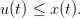(3)

Constraint (3) ensures u(t) is feasible, that is, such that the dam volume remains greater or equal to its minimum value x (remember that any inﬂow w(t) is nonnegative).

#### 1.2 Criterion: intertemporal payoﬀ

The manager problem is one of payoﬀ maximization. At each time t, the turbinated water u(t) produces electricity which is sold on markets at a price p(t). The associated ﬁnancial income is modeled by a linear function L(t,u) = p(t)u, leading to the instantaneous payoﬀ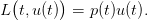(4)

Moreover, the volume x(tf) remaining in the dam at the horizon tf is valued (this is the so-called ﬁnal value of water) using a quadratic function K, leading to the ﬁnal payoﬀ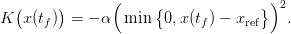(5)

Here, α is a coeﬃcient and xref is a given reference volume for the dam at horizon tf. The ﬁnal payoﬀ is negative if and only if x(tf) < xref. From ti to tf, the payoﬀs sum up to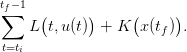(6)

The sequence of pricesp(ti),,p(tf 1)is supposed to be deterministic, hence known in advance at initial time ti where the optimization problem is posed.

#### 1.3 Probabilistic model for water inﬂows

A water inﬂow scenario is a sequence of inﬂows in the dam from time ti up to tf 1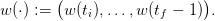(7)

We model water inﬂow scenarios using a sequence of random variables with a known joint probability distribution such that

• the random variablesw(ti),,w(tf 1)are independent,
• each random variable w(t) follows a uniform probability distribution2 on the ﬁnite set 𝕎(t):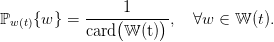(8)

Notice that the random variablesw(ti),,w(tf 1)are independent, but that they are not identically distributed. Independence is a key assumption to obtain the dynamic programming equation (18) with state x.

#### 1.4 Numerical data

Time. We consider a montly management (the interval [t,t + 1[ represents one month) over one year, so that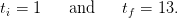(9)

State and control. Concerning the dam volume and the turbinated water, we consider the following bounds: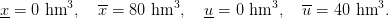(10)

We assume that the dam volume is discretized using a stepsize δx = 2 hm3, whereas the stepsize used for discretizing the control is δu = 8 hm3 (multiple of δx). Accordingly, the sets containing the possible values of the state and the control are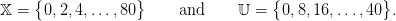The water volume in the dam at time ti is known and equal to xi = 40 hm3, and the reference volume used to deﬁne the ﬁnal payoﬀ K in (5) is xref = 40 hm3.

Prices scenario. The price scenariop(ti),,p(tf 1), known in advance, is shown in Figure 1.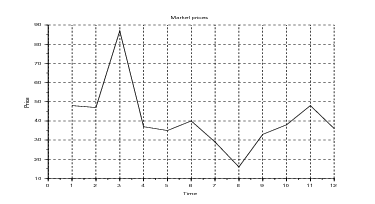Figure 1: Prices trajectory for valuing the turbinated water

Water inﬂow scenarios. At each time t, the range 𝕎(t) of the water inﬂow w(t) is obtained by discretizing an interval centered around a given mean value (see Figure 2). Each interval is discretized using a stepsize δw = 2 hm3 (multiple of δx). Consider for example time t = 1: the minimum (resp. maximum) inﬂow value is w(1) = 12 hm3 (resp. w(1) = 28 hm3), so that the ﬁnite support 𝕎(1) of the (uniform) probability distribution associated with the random variable w(1) is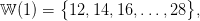the probability weight of each element w 𝕎(1) being 19.

Knowing the probability distribution, it is easy to draw water inﬂow scenarios since we assumed that the random variables w(t) are independent.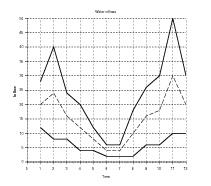Figure 2: Inﬂows probability laws support (left) and some associated scenarios (right)

Scilab code. The following Scilab code contains the data and macros corresponding to the numerical example described in §1.4.

//------------------------------
// PROBLEM DATA
//------------------------------

// Time characteristics
// --------------------

// Time horizon
Ti=1;// Don't change this value!
Tf=13;

// Dam characteristics
// -------------------

xmin=0;
xmax=80;
xini=40;
xref=40;

// Dam discretization
xdlt=2;// Integer: xmin, xmax and xini are multiples of xdlt
Nx=((xmax-xmin)/xdlt)+1;

// Control characteristics
// -----------------------

umin=0;// Don't change this value!
umax=40;

// Control discretization
udlt=8;// Integer, multiple of xdlt: umin and umax are multiples of udlt
Nu=((umax-umin)/udlt)+1;

// Electricity prices
// ------------------

prices=[48.0,47.0,87.0,37.0,35.0,40.0,29.0,16.0,33.0,38.0,48.0,36.0];

// Inflows characteristics
// -----------------------

// Inflow discretization
wdlt=2;// Integer, multiple of xdlt

// Inflow mean values and maximal variations
wexp=[20.0,24.0,16.0,12.0,08.0,04.0,04.0,10.0,16.0,18.0,30.0,20.0];
wect=[08.0,16.0,08.0,08.0,04.0,02.0,02.0,08.0,10.0,12.0,20.0,10.0];

// Probability laws of the inflows (uniform law with finite support)
wlaws=list();
plaws=list();
for t=Ti:Tf-1 do
wlaws(t)=[(wexp(t)-wect(t)):wdlt:(wexp(t)+wect(t))];
plaws(t)=ones(wlaws(t))/length(wlaws(t));
end

// Macro generating Ns inflow scenarios
function wscenarios=inflow_scenarios(Ns,wlaws,plaws)
// Random number generator
grand("setgen","clcg2");
grand("setsd",12345,67890);

// Scenario generation
wscenarios=zeros(Ns,Tf-1);
for t=Ti:Tf-1 do
// Probability law at t
wlawt=wlaws(t);
plawt=plaws(t);
// Probability transition matrix for uniform sampling
// We use grand(...,"markov",...,...) to generate independent sequences
proba=ones(length(plawt),1)*plawt;
samples=grand(Ns,"markov",proba,1);
wscenarios(:,t)=wlawt(samples)';
end
endfunction

Question 1

a)
 Copy this code into a ﬁle DamManagement.sce and check that it corresponds to the data given in §1.4.
b)
 Launch Scilab and execute the ﬁle DamManagement.sce. Use the macro inflow_scenarios to generate 100 scenarios of water inﬂow. Plot the ﬁrst 20 scenarios and compare them to the ones given in Figure 2.

### 2 Simulation and evaluation of given policies

In order to simulate (and then optimize) the dam behavior, we need to have the Scilab macros computing the dynamics (1) and the payoﬀs (4) and (5).

//------------------------------
// DAM MACROS
//------------------------------

function xn=dynamics(t,x,u,w)
// Dynamics of the dam
xn=min(xmax,x-u+w);
endfunction

function payoff=instant_payoff(t,u)
// Instantaneous payoff at time t
payoff=prices(t)*u;
endfunction

function payoff=final_payoff(x)
// Final payoff at horizon
payoff=-min(0,x-xini) .^2;
endfunction

Question 2  Copy the above Scilab code at the end of the ﬁle DamManagement.sce and check that it corresponds to the expressions (1)(4)(5). Notice that these macros are written in such a way that they may be fed either with scalar values or with vector values. This feature will be be used for eﬃciently simulating a bunch of inﬂow scenarios (see Question 3).

#### 2.1 Evaluation of a turbining policy

An admissible policy γ : 𝕋 × 𝕏 𝕌 assigns a turbinated water amount u = γ(t,x) 𝕌 to any time t 𝕋 and to any dam volume x 𝕏, while respecting constraint (3), that ias,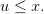Hence, by (2), we obtain that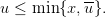Given an admissible policy γ and given an inﬂow scenario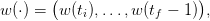(11)

we are able to build a dam volume trajectory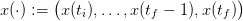(12)

and a turbinated water trajectory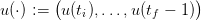(13)

produced by the “closed-loop” dynamics initialized at the initial time ti by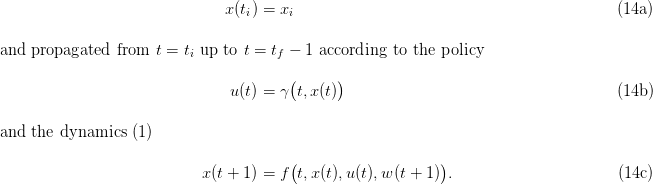We also obtain the payoﬀ associated to the inﬂow scenario w()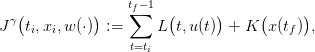(15)

where x() and u() are given by (14c). The expected payoﬀ associated with the policy γ is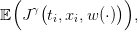(16)

where the expectation 𝔼 is taken with respect to the product probability , whose marginals are given by (8). The true expected value (16) is diﬃcult to compute,3 and we evaluate it by the Monte Carlo method using Ns inﬂow scenariosw1(),,wNs():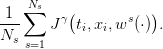(17)

By the law of large numbers, the mean payoﬀ (17) is a “good” approximation of the expected payoﬀ(16) if the number of scenarios is “large enough”.

We propose the following Scilab code in order to evaluate the mean payoﬀ associated to a policy given by the Scilab macro policy.

//------------------------------
// SCENARIO BASED SIMULATOR
//------------------------------

function [xscenarios,uscenarios,cscenarios]=simulation(wscenarios,policy)
// Initialization
xscenarios=zeros(Ns,Tf);// used to store the state trajectories
uscenarios=zeros(Ns,Tf-1);// used to store the control trajectories
cscenarios=zeros(Ns);// used to store the payoff values

// Simulation in forward time
xscenarios(:,1)=xini*ones(Ns,1);
for t=Ti:Tf-1 do
x=xscenarios(:,t);
u=policy(t,x);
w=wscenarios(:,t);
xn=dynamics(t,x,u,w);
cscenarios=cscenarios+instant_payoff(t,u);
xscenarios(:,t+1)=xn;
uscenarios(:,t)=u;
end
cscenarios=cscenarios+final_payoff(xn);
printf('\n Mean payoff (Monte Carlo): %f\n',mean(cscenarios));
endfunction

Question 3

a)
 Copy the above Scilab code at the end of the ﬁle DamManagement.sce. Check that it corresponds to the expressions (14c)(15)(17) for a given policy γ (input argument policy of the macro simulation).
b)
 Explain in detail how the macro simulation computes these quantities (see Question 2).

#### 2.2 Simulation of a given policy

In order to test the Scilab macro simulation given above, consider the following code.

//------------------------------
// HEURISTIC POLICY EVALUATION
//------------------------------

function u=heuristic_policy(t,x)
// Heuristic policy
thresmin=xref;
thresmax=xref;
u=(umax*bool2s(x > thresmin))+(umin*bool2s(x <= thresmax));
u=min(u,x);
endfunction

// Scenarios generation
Ns=10000;
wscenarios=inflow_scenarios(Ns,wlaws,plaws);

// Simulation
[xscenarios,uscenarios,cscenarios]=simulation(wscenarios,heuristic_policy);

// Payoff histogram
xset("window",101);
clf();
histplot(50,cscenarios,normalization = %t,style = 5);
xgrid;
xtitle("Payoff distribution");

// State trajectories
xset("window",102);
clf();
plot2d([Ti:Tf],xscenarios(1:20,:)');
xgrid;
xtitle('Dam volume','Time','State');

// Control trajectories
xset("window",103);
clf();
plot2d([Ti:Tf-1],uscenarios(1:20,:)');
xgrid;
xtitle('Turbined water','Time','Control');

Question 4

(a)
 Explain the control policy induced by the Scilab macro heuristic_policy. Copy the relevant part of the above Scilab code at the end of DamManagement.sce, then generate 10,000 inﬂow scenarios and simulate the policy heuristic_policy along them.
(b)
 Copy the relevant part of the above Scilab code at the end of DamManagement.sce, then plot some trajectories corresponding to the dam volume and to the turbinated water obtained using this policy, and provide a histogram of the payoﬀ distribution. Comment the ﬁgures thus obtained.

We now want to design new policies, writing Scilab macros following the model given by the macro below:

function u=my_policy(t,x)
u=max(umin,min(WRITE_A_FORMULA_HERE,min(x,umax)));
endfunction

Question 5

(a)
 Explain why we use the expression max(umin,min( ..... , min(x,umax))) in the macro my_policy above.
(b)
[1+1+2] Write the Scilab code of the following policies:
• always turbinate at the maximum possible (myopic),
• turbinate a fraction of the maximum possible,

and evaluate them thanks to the scenario based simulator.

(c)
 Propose a policy which depends on the prices knowledge, and explain how you designed this policy.
(d)
 Evaluate this last policy, and compare it to the ones designed in item (b).

### 3 Resolution by the stochastic dynamic programming approach

In order to compute the optimal turbinated water policy, we provide the stochastic dynamic programming (or Bellman) equation associated with the problem of maximizing the expected payoﬀ (16), as well as the corresponding Scilab code.

#### 3.1 Stochastic dynamic programming equation

Since the water inﬂows w(t) are supposed to be independent random variables, Dynamic Programming applies and the equation associated with the problem of maximizing the expected payoﬀ  (16) writes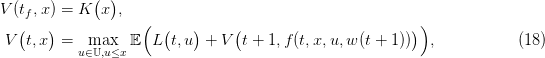for t varying from tf 1 to ti. The expectation 𝔼 is taken with respect to the marginal probability distribution w(t+1) given by (8), that is,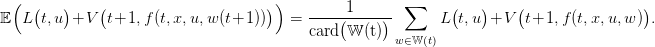A diﬃculty when programming the Bellman equation is that we need to manipulate both the values (t,x) of the time and of the state, and the associated indices (t,i) in the table storing the values of the function V (t,x). In our application, the treatment of index t is obvious because t 𝕋 = {ti,ti + 1,,tf}, but we need a tool performing the conversion for the volume. The following Scilab macro state_index returns the index i associated to an element x in the ﬁnite set 𝕏 containing the possible values of the dam volume.

function i=state_index(x)
// Index associated with a volume value
i=dsearch(x,[xmin:xdlt:xmax],'d');
if i==0 then
printf('\n Invalid dam volume: %f\n',x);
end
endfunction

Question 6  Copy the above Scilab code at the end of the ﬁle DamManagement.sce. Explain in detail how the macro works.

#### 3.2 Scilab code for the dynamic programming equation

We provide the following code in order to compute the Bellman function.

//-------------------------------
// STOCHASTIC DYNAMIC PROGRAMMING
//-------------------------------

// Initialization
Vbell=-ones(Tf,Nx)*%inf;// Used to store the optimal payoff functions
Ubell=ones(Tf-1,Nx)*%inf;// Used to store the optimal controls

// SDP computation by backward induction
function [Vbell,Ubell]=sdp_solve()
// Bellman function at final time
payoff=final_payoff([xmin:xdlt:xmax]);
Vbell(Tf,:)=payoff';
// Loop backward in time
for t=Tf-1:-1:Ti do
wlawt=wlaws(t);
plawt=plaws(t);
Nw=length(wlawt);
for x=xmin:xdlt:xmax do
i=state_index(x);
for u=[umin:udlt:min(x,umax)] do
ctot=0;
for k=1:Nw do
w=wlawt(k);
p=plawt(k);
xn=dynamics(t,x,u,w);
in=state_index(xn);
ctot=ctot+(p*Vbell(t+1,in));
end
ctot=ctot+instant_payoff(t,u);
if ctot > Vbell(t,i) then
Vbell(t,i)=ctot;
Ubell(t,i)=u;
end
end
end
end
endfunction

Question 7

(a)
 Copy the above Scilab code at the end of the ﬁle DamManagement.sce. Explain in detail why the macro sdp_solve corresponds to the computation of the Dynamic Programming equation (18).
(b)
 Execute the macro sdp_solve, which generates the table Vbell containing the values of the Bellman function and the table Ubell containing the values of the optimal controls. Display the value of the Bellman function at (ti,xi).
(c)
 Plot diﬀerent Bellman functions and comment on their shape.

#### 3.3 Simulation of the optimal policy

Consider the following Scilab macro.

function u=optimal_policy(t,x)
i=state_index(x);
u=Ubell(t,i)';
endfunction

Question 8

(a)
 Explain why the control policy induced by the Scilab macro optimal_policy is optimal.
(b)
 Generate 10,000 water inﬂow scenarios using the macro simulation and simulate the policy optimal_policy along them. Plot some trajectories corresponding to the dam volume and to the turbinated water, obtained using this policy.
(c)
 Provide a histogram of the distribution of the random payoﬀ, and compare the mean payoﬀ (17), with the value V (ti,xi) of the Bellman function evaluated at (ti,xi).
(d)
 Compare these results with those obtained using all previous policies.

Here we assume that the values of optimal control have not be stored in the table Ubell, so that the only outcome of the macro sdp_solve is the table Vbell (Bellman values).

Question 9  Provide a method and the corresponding code to compute the optimal value of the control at time t for a given dam volume x(t) using only the values stored in the table Vbell at time t + 1.

Hint: the optimal control at (t,x(t)) is the arg max of the following optimization problem: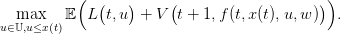(19)

Be careful that your code must accept a vector of volumes as input.

### 4 Possible extensions

In this section, we propose several developments and extensions for the dam management problem.

#### 4.1 Hazard-decision framework

In the hazard-decision framework, the dam manager is supposed to make a decision, here turbining u(t) at the beginning of the period [t,t + 1[, knowing the water inﬂow w(t + 1) in advance.

The Bellman equation is now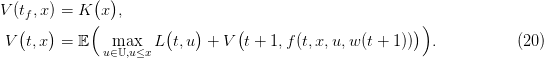Once the value function V (t,x) evaluated and stored for all time t and state x, the optimal control at time t starting from state x and observing the water inﬂow w is the arg max of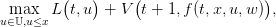(21)

which leads to an optimal policy depending on the triplet (t,x,w).

Question 10

(a)
 Explain the diﬀerence between (20) and (18).
(b)
 Adapt the Scilab macro sdp_solve to solve the hazard-decision Bellman equation (20). We decide not to store the optimal policy in the table Ubell.
(c)
[1+2] Based on (21), write the Scilab code which computes the optimal control as a function of (t,x,w), and use it to obtain by simulation the optimal trajectories of the state and the control as well as the optimal payoﬀ distribution. Compare.

#### 4.2 Computation of a fair ﬁnal value of water

Till now, the gain in leaving water in the dam at the end of the time horizon was arbitrarily ﬁxed. Now, we provide a procedure to estimate a “ fair ﬁnal value of water”, that is, a function x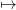K(x) as in (5).

The intuition behind the procedure is that the ﬁnal value of water is the value that a manager would put on the dam, were he to run it from the date tf + 1 to inﬁnity. The ﬁnal value of water is the solution of an inﬁnite horizon maximization problem. The procedure below mimicks an algorithm to ﬁnd a ﬁxed point by iterations.

First, we start with a zero ﬁnal payoﬀ function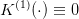and obtain, by backward induction, the Bellman function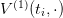at initial time ti. Up to a translation — to account for the fact that an empty dam has zero ﬁnal value of water — we identify the function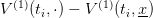with the new ﬁnal value of water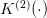. Proceeding along, we expect that this loop converges towards a function xK()(x) which is a good candidate for the ﬁnal value of water.

We design a loop for n = 1,,N, starting with a zero ﬁnal value of water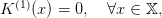then solving the backward Bellman equation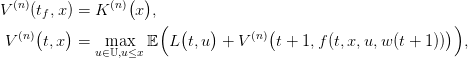and then closing the loop by choosing the new ﬁnal value of water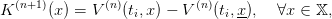(22)

as the Bellman function at the initial time ti after a shift, so that K(n+1)(x) = 0.

Question 11 Implement the iterative procedure described above, and compute and plot the “fair ﬁnal value of water” in the dam.

#### 4.3 Robustness with respect to water inﬂow scenarios

An interesting point, which occurs in practical problems, is the following. In the case where the random variablesw(ti),,w(tf 1)are correlated in time, it is always possible to obtain the marginal probability laws w(t) of each w(t) and then compute the Bellman function as in §3. We thus obtain a feedback policy that would be optimal if the random inﬂow variables were uncorrelated. The loss of optimality may be evaluated by simulating the feedback policy along scenarios drawn using the “true” probability law of the processw(ti),,w(tf 1).

Question 12

(a)
Draw inﬂow scenarios according to an auto-regressive AR(1) process.
(b)
Compute the marginal probability distribution w(t) induced by these scenarios.
(c)
Compute the Bellman solution of the problem using these marginal probability laws and the associated optimal policy.
(d)
Using this policy, run a simulation along scenarios drawn according to the AR(1) process.
(e)
Evaluate the loss of optimality induced by ignoring the correlation in the inﬂow process.

### References

D. P. Bertsekas. Dynamic Programming and Optimal Control. Athena Scientiﬁc, Belmont, Massachusets, second edition, 2000. Volumes 1 and 2.

M. De Lara and L. Doyen. Sustainable Management of Natural Resources. Mathematical Models and Methods. Springer-Verlag, Berlin, 2008.

 L'École des Ponts ParisTech est membre fondateur de L'École des Ponts ParisTech est certifiée